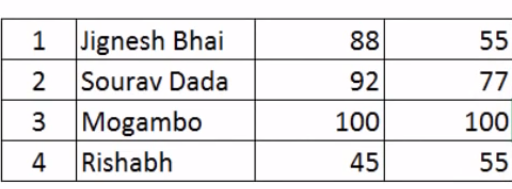# Excel VLOOKUP FormulaIn this blog, we will learn about VLOOKUP Formula in Excel

How to use VLOOKUP in Excel?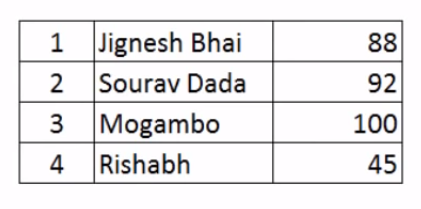I have a table consists of 4 students along with their test scores. Now I want to know the test score of Sourav Dada.

1. Copy the name of Sourav Dada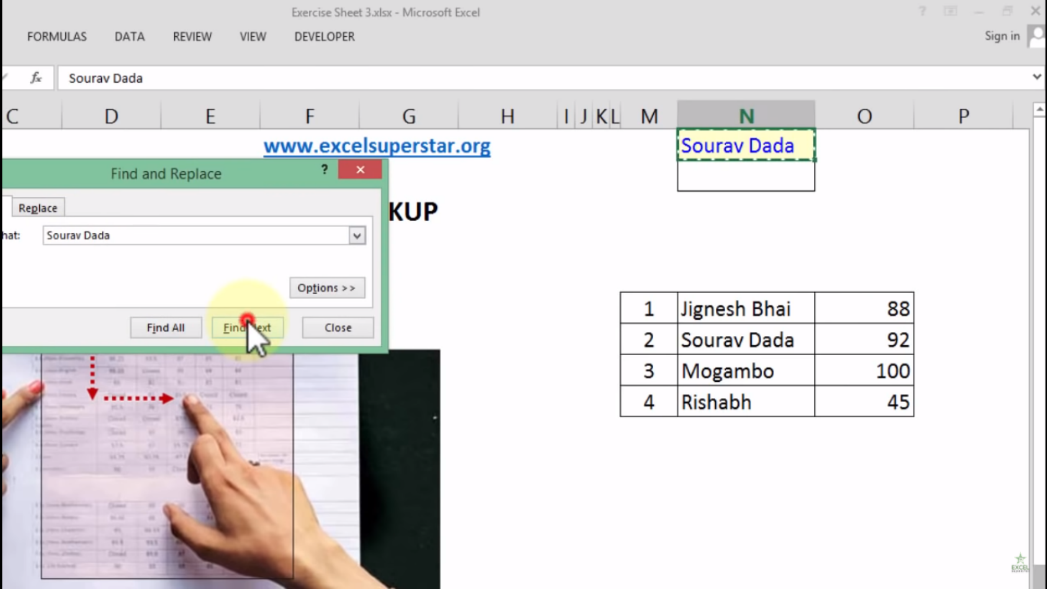2. Then Press Ctrl + F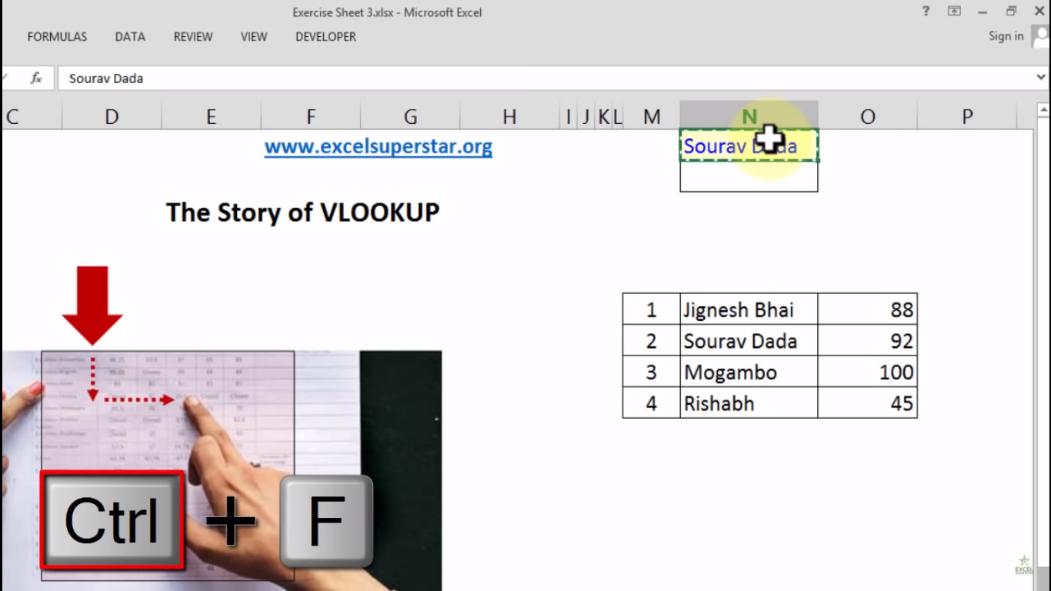3. A Find and Replace Panel will open paste the name on it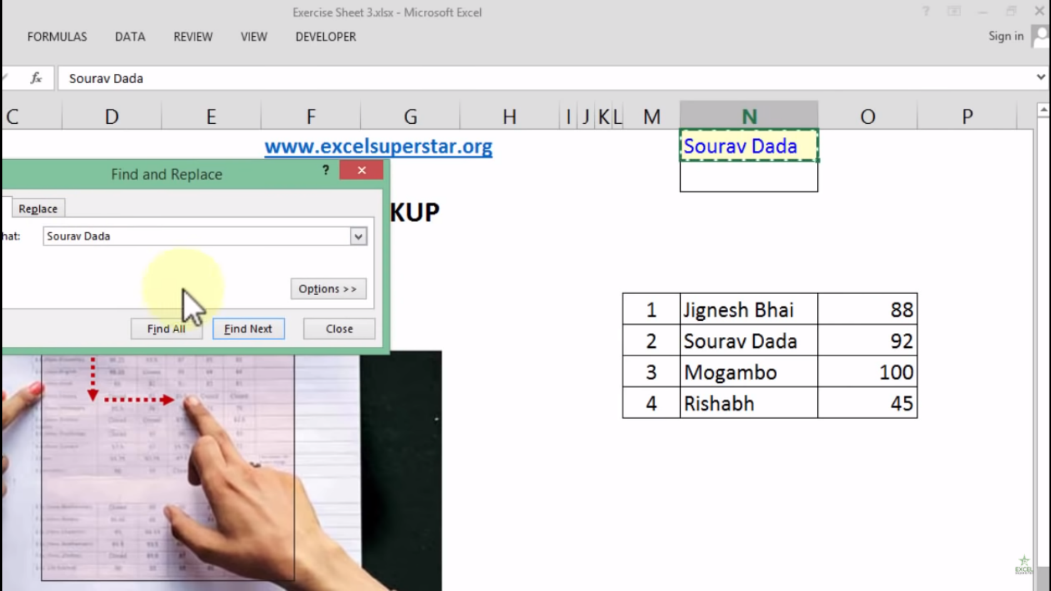4. Click on Find Next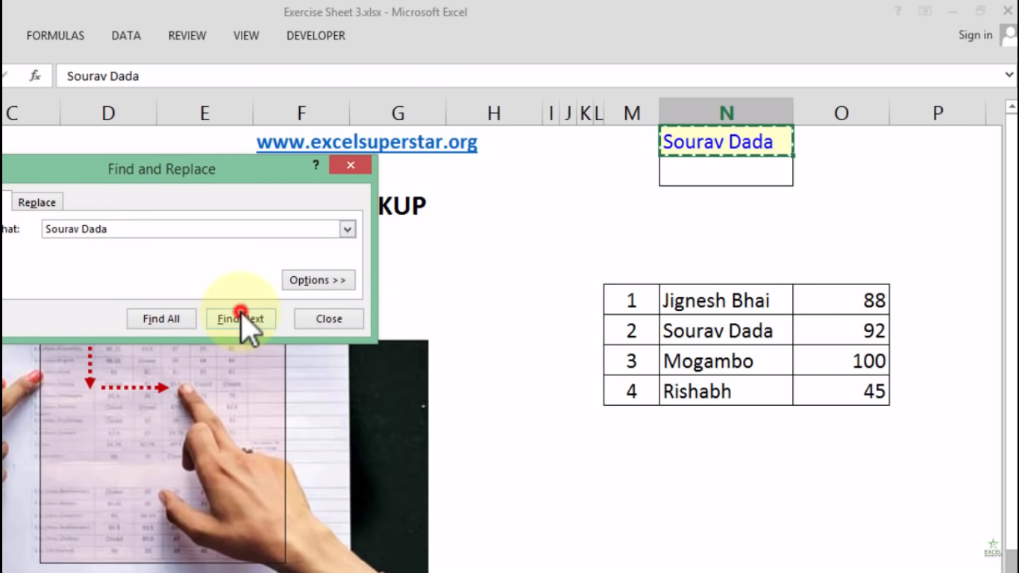5. Lastly, the cursor will navigate and click on your name in order to know the answer

OR

How to apply VLOOKUP formula to find an answer in Excel?

To apply VLOOKUP Formula in Excel, follow the steps given below:

1. Write =vlookup and press the Tab Key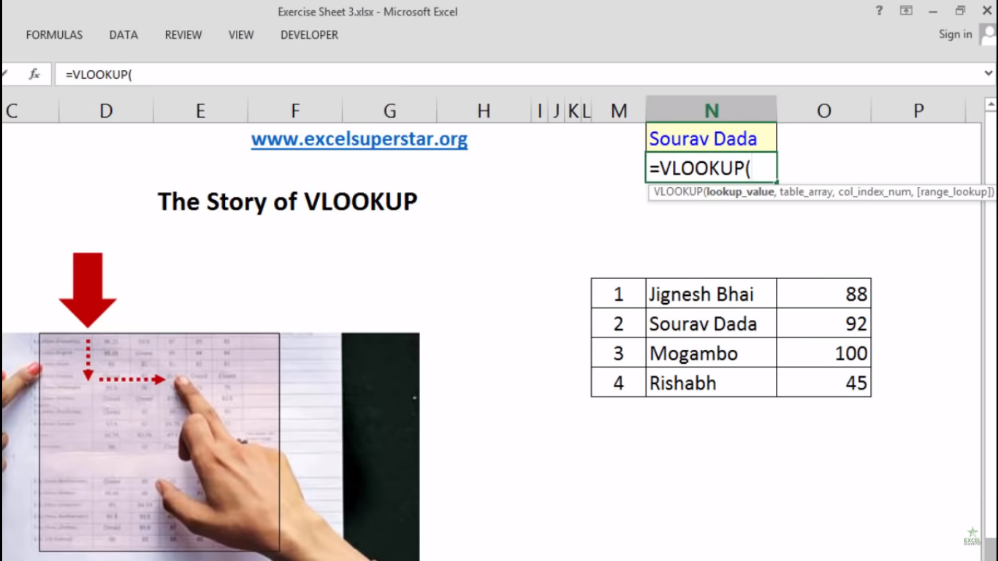The VLOOKUP syntax has the following arguments:

1. value – The value to look for in the first column of a table.

2. table – The table from which to retrieve a value.

3. col_index – The column in the table from which to retrieve a value.

4. range_lookup – [optional] TRUE = approximate match (default). FALSE = exact match.

2. Select the cell from the table =VLOOKUP[N1,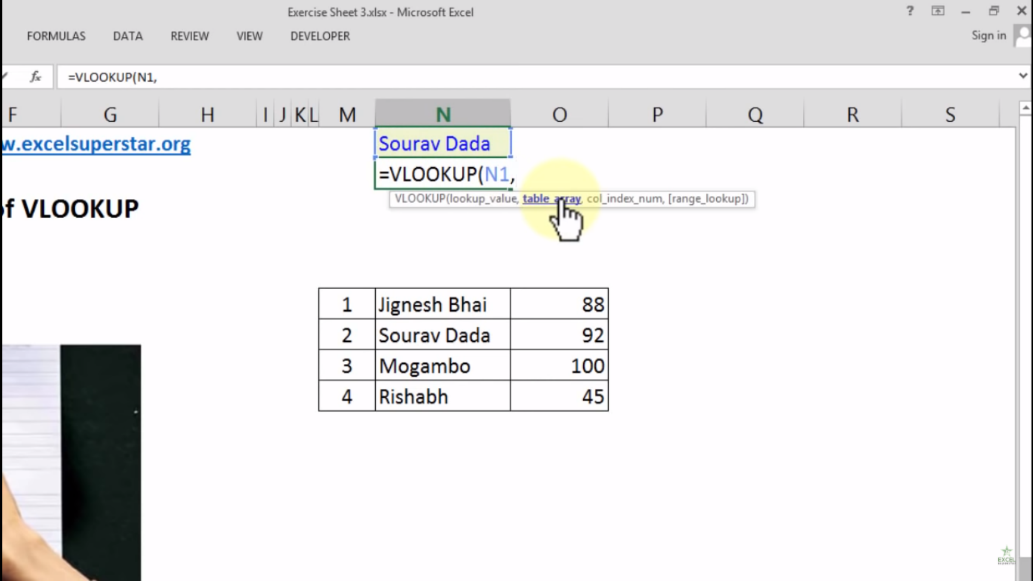3. Select the table array =VLOOKUP[N1,N6:09,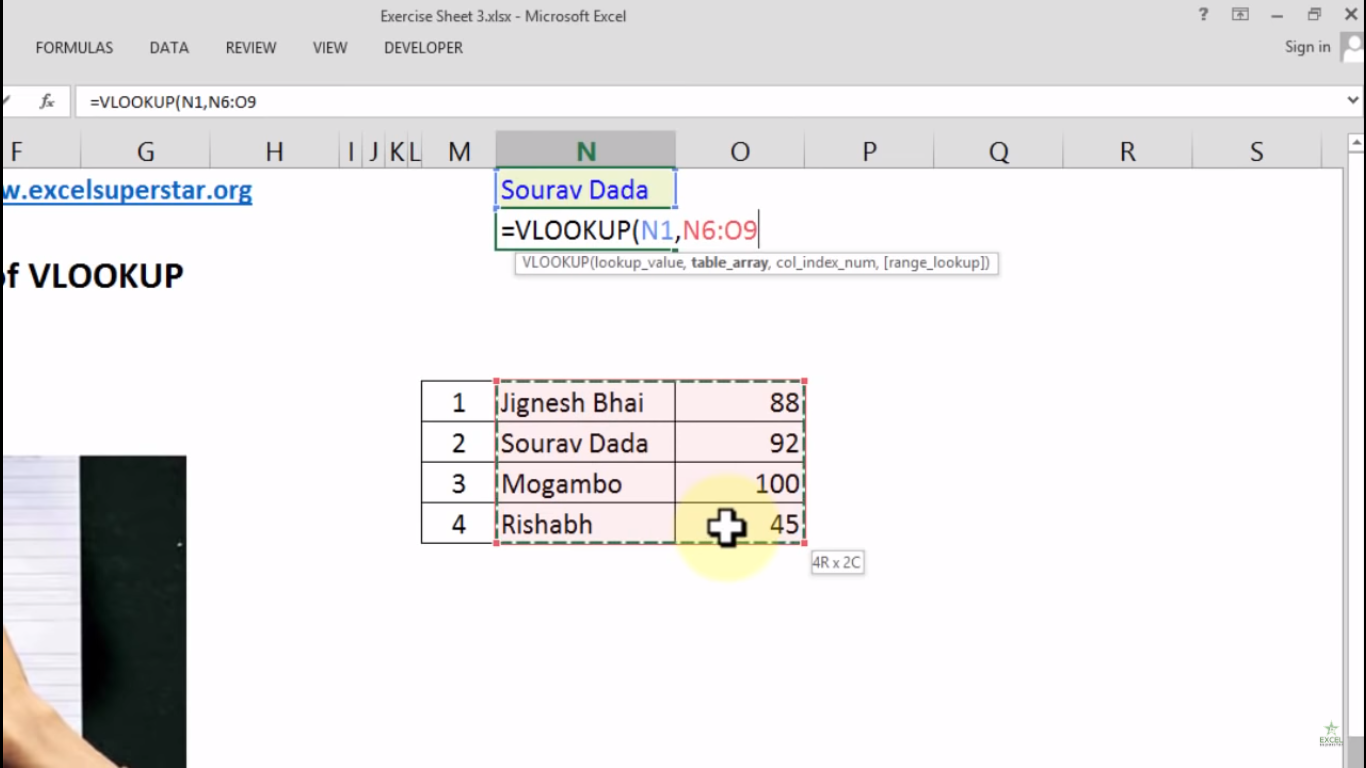4. Write 2 as from column 2 we want to retrieve a value =VLOOKUP[N1,N6:09,2,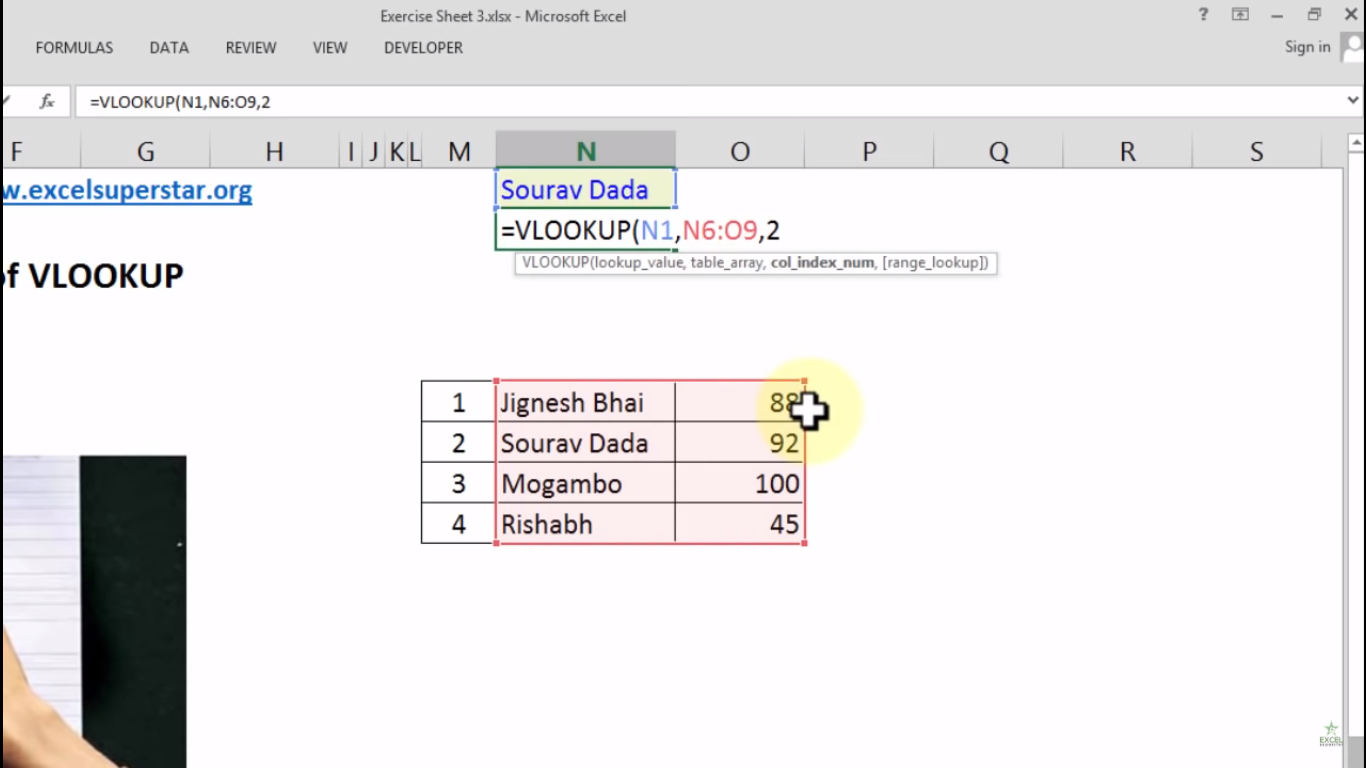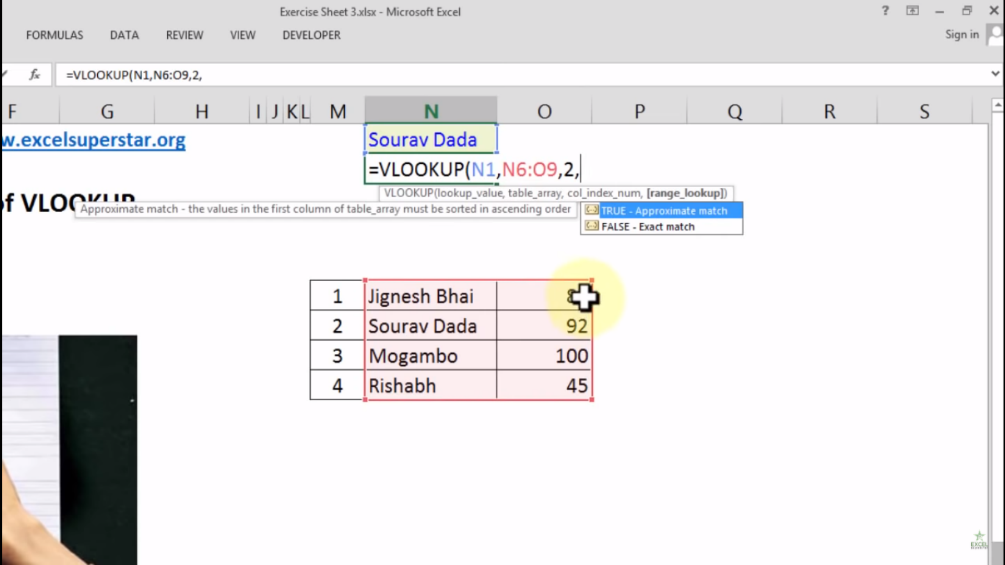5. Select FALSE as an exact match VLOOKUP[N1,N6:09,2, FALSE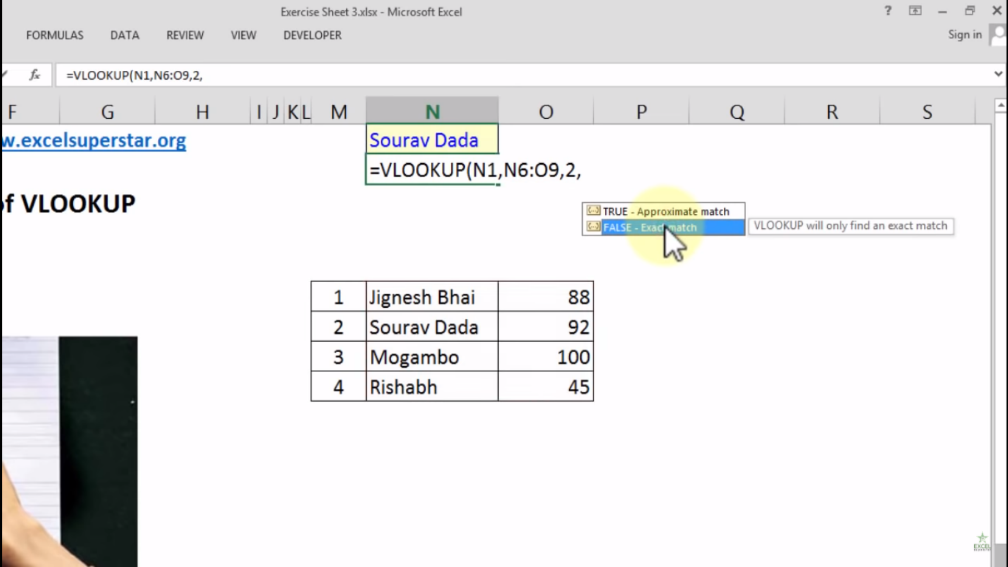6. Close the bracket and press Enter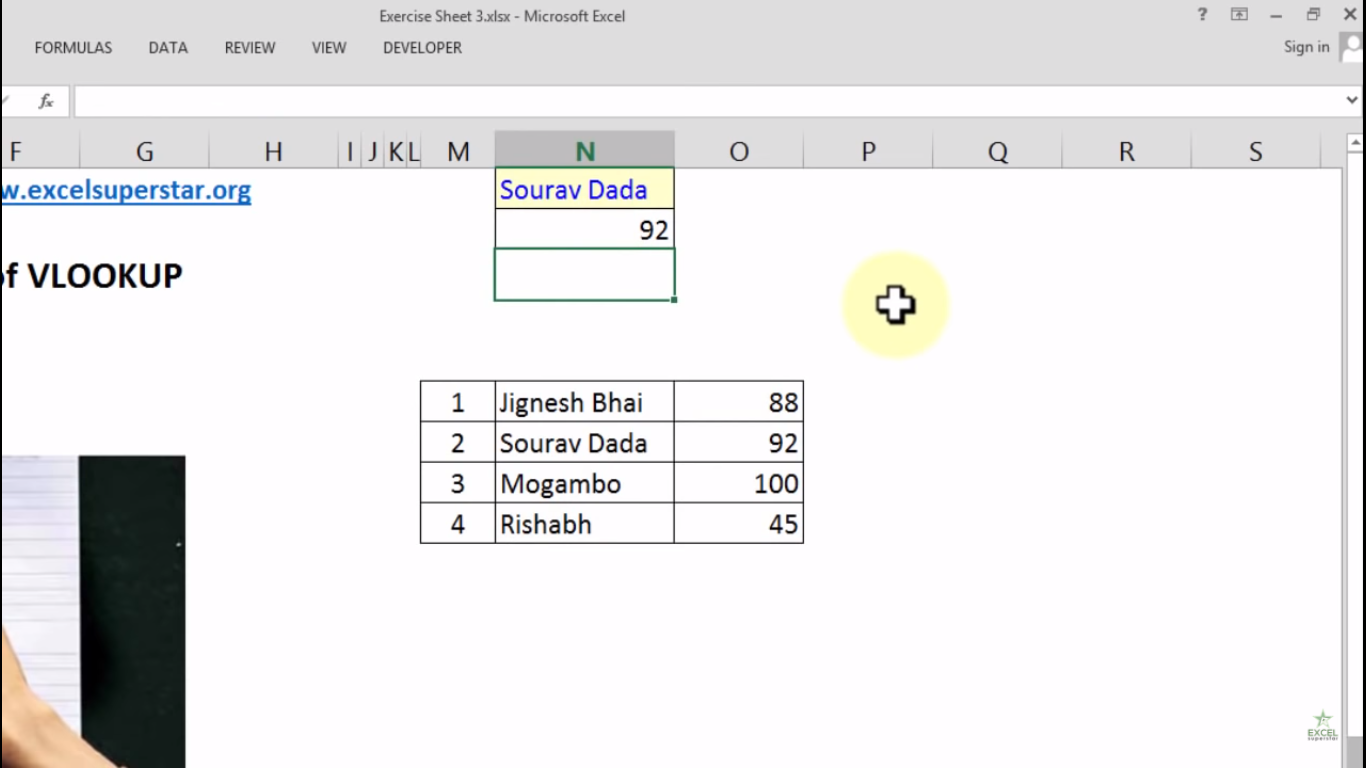BONUS TIP

In this table I have added second column of Subjects. Now I want to find out the test scores scored by students in the second subject